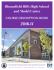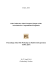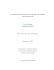# Algebraic Expressions—The Commutative and Associative Properties

## Transcription

Algebraic Expressions—The Commutative and Associative Properties
```Lesson 7
A STORY OF FUNCTIONS
M1
ALGEBRA I
Lesson 7: Algebraic Expressions—The Commutative and
Associative Properties
Student Outcomes

Students use the commutative and associative properties to recognize structure within expressions and to
prove equivalency of expressions.
Classwork
Exercises 1–4 (15 minutes)
Have students discuss the following four exercises in pairs. Discuss the answers as a class.
Exercise 1
Suzy draws the following picture to represent the sum  + :
Ben looks at this picture from the opposite side of the table and says, “You drew  + .”
Explain why Ben might interpret the picture this way.
Ben read the picture from his left to his right on his side of the table.
Exercise 2
Suzy adds more to her picture and says, “The picture now represents ( + ) + .”
How might Ben interpret this picture? Explain your reasoning.
Reading from right to left, the solution would be ( + ) + . Make sure students have parentheses around  + .
Lesson 7:
Algebraic Expressions—The Commutative and Associative Properties
This work is derived from Eureka Math ™ and licensed by Great Minds. ©2015 -Great Minds. eureka math.org
ALG I-M1-TE-1.3.0-05.2015
76
Lesson 7
A STORY OF FUNCTIONS
M1
ALGEBRA I
Exercise 3
Suzy then draws another picture of squares to represent the product  × . Ben moves to the end of the table and says,
“From my new seat, your picture looks like the product  × .”
What picture might Suzy have drawn? Why would Ben see it differently from his viewpoint?
Squares should be arranged in a grid. If a student responds that Suzy made  rows of , then Ben’s viewpoint would be
rows of . Students should understand that Ben is seated to Suzy’s left or right now, not across from her. Some students
may need scaffolding here—have them physically move to see the different viewpoint.
Exercise 4
Draw a picture to represent the quantity ( × ) ×  that also could represent the quantity ( × ) ×  when seen from
a different viewpoint.
Student solutions could vary here. Students may consider representing the problem as a  by  by  rectangular box.
When viewed from different faces, the different expressions appear. With the  by  rectangle viewed as its base, the
volume of the box might be computed as ( × ) × . But with the  by  rectangle viewed as its base, its volume would
be computed as ( × ) × . Some students will likely repeat the  ×  pattern  times in a row. This diagram viewed
from the end of the table would be  dots repeated  times arranged in  columns.
Ask students a series of questions of the following type:

1
2
Could the ideas developed in Exercises 1 and 2 be modified so as to explain why 2 +
3
19
1
+2 ?
2
3
should equal
19

Or that �(−6562.65) + (−9980.77)� + 22 should equal �22 + (−9980.77)� + (−6562.65)?

Is it possible for a rectangle or a rectangular box to have a negative side length?


1
1
should equal +

√2?
Could the ideas developed in Exercises 3 and 4 be used to show that (−3) × �
or that ( × 17.2) × �−
16
16
� should equal ��− � × 17.2� × ?
5
5
Lesson 7:
1
�7
Algebraic Expressions—The Commutative and Associative Properties
This work is derived from Eureka Math ™ and licensed by Great Minds. ©2015 -Great Minds. eureka math.org
ALG I-M1-TE-1.3.0-05.2015
� should equal �
1
� × (−3)
√7
77
Lesson 7
A STORY OF FUNCTIONS
M1
ALGEBRA I
Next, have students review the four properties of arithmetic provided in the student materials and ask the following:
Four Properties of Arithmetic:
THE COMMUTATIVE PROPERTY OF ADDITION: If  and  are real numbers, then  +  =  + .
THE ASSOCIATIVE PROPERTY OF ADDITION: If , , and  are real numbers, then ( + ) +  =  + ( + ).
THE COMMUTATIVE PROPERTY OF MULTIPLICATION: If  and  are real numbers, then  ×  =  × .
THE ASSOCIATIVE PROPERTY OF MULTIPLICATION: If , , and  are real numbers, then () = ().

Can you relate each of these properties to one of the previous exercises?

Exercise 1 connects with the commutative property of addition.

Exercise 2 connects with the associative property of addition. (Students might mention that the
commutative property of addition is also relevant to this exercise. This will be discussed fully in
Exercise 5.)

Exercise 3 connects with the commutative property of multiplication.

Exercise 4 connects the associative property of multiplication. (Students might mention that the
commutative property of multiplication is also relevant to this exercise. This will be discussed fully in
Exercise 6.)
Point out that the four opening exercises suggest that the commutative and associative properties of addition and
multiplication are valid for whole numbers and probably apply to all real numbers as well. However, there is a weakness
in the geometric models since negative side lengths and areas are not meaningful. We choose to believe these
properties hold for all real numbers, including negative numbers.
Exercise 5 (10 minutes)
Exercise 5
Viewing the diagram below from two different perspectives illustrates that ( + ) +  equals  + ( + ).
Is it true for all real numbers , , and  that ( + ) +  should equal ( + ) + ?
(Note: The direct application of the associative property of addition only gives ( + ) +  =  + ( + ).)
To answer this exercise with the class, create a flow diagram on the board as follows. This flow diagram will show how
one can apply both the commutative and associative properties to prove the equivalence of these two expressions.
Have students copy this work into their handouts.
Start by showing the application of each property on the expression ( + ) + .
Lesson 7:
Algebraic Expressions—The Commutative and Associative Properties
This work is derived from Eureka Math ™ and licensed by Great Minds. ©2015 -Great Minds. eureka math.org
ALG I-M1-TE-1.3.0-05.2015
78
Lesson 7
A STORY OF FUNCTIONS
M1
ALGEBRA I
Here A represents an application of the associative property and C an application of the commutative property. Be sure
students understand the application of the commutative property shown here.
Point out that we can extend this diagram by applying the commutative and associative properties to the new
expressions in the diagram.
Note that there are multiple branches and options for extending this diagram. Direct the students to discover options
that will chart a path from ( + ) +  to ( + ) + . Two possible paths are as follows:
Lesson 7:
Algebraic Expressions—The Commutative and Associative Properties
This work is derived from Eureka Math ™ and licensed by Great Minds. ©2015 -Great Minds. eureka math.org
ALG I-M1-TE-1.3.0-05.2015
79
Lesson 7
A STORY OF FUNCTIONS
M1
ALGEBRA I
Choose one of the paths in the flow diagram and show on the board how to write it as a mathematical proof of the
statement that ( + ) +  and ( + ) +  are equivalent expressions. For example, for the lower of the two paths
shown, write the following:
( + ) +
=  + ( + )
=  + ( + )
= ( + ) +
commutative property
commutative property
associative property
Exercise 6 (5 minutes)
Exercise 6
Draw a flow diagram and use it to prove that () = () for all real numbers , , and .
Here is the start of the diagram.
Students will likely realize the answer here is completely analogous to the solution to the previous exercise.
()
= ()
= ()
= ()
commutative property
commutative property
associative property
Have students complete Exercises 7 and 8.
Lesson 7:
Algebraic Expressions—The Commutative and Associative Properties
This work is derived from Eureka Math ™ and licensed by Great Minds. ©2015 -Great Minds. eureka math.org
ALG I-M1-TE-1.3.0-05.2015
80
Lesson 7
A STORY OF FUNCTIONS
M1
ALGEBRA I
Exercise 7 (5 minutes)
Exercise 7
Use these abbreviations for the properties of real numbers, and complete the flow diagram.
+ for the commutative property of addition
× for the commutative property of multiplication
+ for the associative property of addition
× for the associative property of multiplication
Exercise 8 (2 minutes)
Exercise 8
Let , , , and  be real numbers. Fill in the missing term of the following diagram to show that �( + ) + � +  is
sure to equal  + � + ( + )�.
+ �( + ) + �
Lesson 7:
Algebraic Expressions—The Commutative and Associative Properties
This work is derived from Eureka Math ™ and licensed by Great Minds. ©2015 -Great Minds. eureka math.org
ALG I-M1-TE-1.3.0-05.2015
81
Lesson 7
A STORY OF FUNCTIONS
M1
ALGEBRA I

This example illustrates that it is possible to prove, through repeated use of the associative property, that any
two arrangements of parentheses around a given sum are equivalent expressions. For this reason it is deemed
unnecessary to place parentheses among a sum of terms. (Present the following on the board.)
�( + ) + � +

+ ( + ( + ))
( + ) + ( + )
+  +  +

From now on, we will accept this as common practice. In presenting a proof, writing the following:
� + ( + ) + ( + 6)� = �( + ) + ( + ) + 6�
by the associative property
OR
+  +  +  =  + ( + ) +
MP.8
by the associative property
for instance, is accepted.
The same holds for a product of symbols. Repeated application of the associative property of multiplication
establishes the equivalency of �()� and �()�, for example, and these can both be written simply as
.
Closing (5 minutes)

Throughout this lesson, we have used symbols for numbers and symbols for placeholders for numbers to create
expressions. Let us now formalize these notions with definitions.
NUMERICAL SYMBOL: A numerical symbol is a symbol that represents a specific number.

For example, , , , , , −, −. , ,  are numerical symbols used to represent specific points on the real
number line.
VARIABLE SYMBOL: A variable symbol is a symbol that is a placeholder for a number.
It is possible that a question may restrict the type of number that a placeholder might permit (e.g., integers only or
positive real numbers).

The following is a general definition:
ALGEBRAIC EXPRESSION: An algebraic expression is either

1.
A numerical symbol or a variable symbol, or
2.
The result of placing previously generated algebraic expressions into the two blanks of one of the four operators
((__) + (__), (__) − (__), (__) × (__), (__) ÷ (__)) or into the base blank of an exponentiation with exponent that is
a rational number.
For example,  and 3 are algebraic expressions, and from that we can create the expression  + 3 by placing
each into the blanks of the addition operator. From there, we can create the expression ( + 3) by placing
and  + 3 into the blanks of the multiplication operator, and so on. According to this general definition, we can
(+3) −2
also create expressions of the form �
Lesson 7:
3 2
� .
Algebraic Expressions—The Commutative and Associative Properties
This work is derived from Eureka Math ™ and licensed by Great Minds. ©2015 -Great Minds. eureka math.org
ALG I-M1-TE-1.3.0-05.2015
82
Lesson 7
A STORY OF FUNCTIONS
M1
ALGEBRA I

Our notion of two expressions being equivalent has also been vague. We can now pinpoint what we mean:
Two algebraic expressions are equivalent if we can convert one expression into the other by repeatedly applying the
commutative, associative, and distributive properties and the properties of rational exponents to components of the first
expression.

Some final jargon:
NUMERICAL EXPRESSION: A numerical expression is an algebraic expression that contains only numerical symbols (no variable
symbols), which evaluate to a single number.
The expression  ÷  is not a numerical expression.
EQUIVALENT NUMERICAL EXPRESSIONS: Two numerical expressions are equivalent if they evaluate to the same number.

For example, although we formally proved (3 + 4) + 2 equals (2 + 4) + 3 through the commutative and
associative properties, it is more reasonable to note that they both evaluate to 9.
Note that  +  +  and  ×  × , for example, are equivalent numerical expressions (they are both ), but  +  +
and  ×  ×  are not equivalent expressions.
Lesson Summary
The commutative and associative properties represent key beliefs about the arithmetic of real numbers. These
properties can be applied to algebraic expressions using variables that represent real numbers.
Two algebraic expressions are equivalent if we can convert one expression into the other by repeatedly applying
the commutative, associative, and distributive properties and the properties of rational exponents to components
of the first expression.
Exit Ticket (3 minutes)
Lesson 7:
Algebraic Expressions—The Commutative and Associative Properties
This work is derived from Eureka Math ™ and licensed by Great Minds. ©2015 -Great Minds. eureka math.org
ALG I-M1-TE-1.3.0-05.2015
83
Lesson 7
A STORY OF FUNCTIONS
M1
ALGEBRA I
Name ___________________________________________________
Date____________________
Lesson 7: Algebraic Expressions—The Commutative and
Associative Properties
Exit Ticket
Write a mathematical proof of the algebraic equivalence of () and ().
Lesson 7:
Algebraic Expressions—The Commutative and Associative Properties
This work is derived from Eureka Math ™ and licensed by Great Minds. ©2015 -Great Minds. eureka math.org
ALG I-M1-TE-1.3.0-05.2015
84
Lesson 7
A STORY OF FUNCTIONS
M1
ALGEBRA I
Exit Ticket Sample Solution
Write a mathematical proof of the algebraic equivalence of () and ().
() = ()
= ()
associative property
commutative property
Problem Set Sample Solutions
1.
The following portion of a flow diagram shows that the expression  +  is equivalent to the expression  + .
Fill in each circle with the appropriate symbol: Either + (for the commutative property of addition) or × (for the
commutative property of multiplication).
2.
Fill in the blanks of this proof showing that ( + )( + ) is equivalent to  +  + . Write either
commutative property, associative property, or distributive property in each blank.
( + )( + )
= ( + ) + ( + ) ×
= ( + ) + ( + ) ×
= ( + ) + ( + )
distributive property
commutative property
commutative property

distributive property

distributive property
=  +  ×  + ( + )

=  +  + ( + )
=  +  +  +

=  + ( + ) +
commutative property
associative property

=  +  +
Lesson 7:
Algebraic Expressions—The Commutative and Associative Properties
This work is derived from Eureka Math ™ and licensed by Great Minds. ©2015 -Great Minds. eureka math.org
ALG I-M1-TE-1.3.0-05.2015
85
Lesson 7
A STORY OF FUNCTIONS
M1
ALGEBRA I
3.
Fill in each circle of the following flow diagram with one of the letters: C for commutative property (for either
addition or multiplication), A for associative property (for either addition or multiplication), or D for distributive
property.
4.
What is a quick way to see that the value of the sum  +  +  +  is ?
+  +  +  = ( + ) + ( + ) =  +
5.
a.
b.
If  =  and  =

, what is the value of the product  ×  ×  × ?

×  ×  ×  = ()() =
Give some indication as to how you used the commutative and associative properties of multiplication to
evaluate  ×  ×  ×  in part (a).
×  ×  ×  =  ×  ×  ×  by two applications of the commutative property of multiplication and
×  ×  ×  = ()() by the associative property of multiplication.
c.
Did you use the associative and commutative properties of addition to answer Problem 4?
Yes, they were used in an analogous manner.
Lesson 7:
Algebraic Expressions—The Commutative and Associative Properties
This work is derived from Eureka Math ™ and licensed by Great Minds. ©2015 -Great Minds. eureka math.org
ALG I-M1-TE-1.3.0-05.2015
86
Lesson 7
A STORY OF FUNCTIONS
M1
ALGEBRA I
6.
The following is a proof of the algebraic equivalency of () and  . Fill in each of the blanks with either the
statement commutative property or associative property.
()
=  ∙  ∙
= ( × )( × )
associative property
=  ∙ ( × ) ∙
associative property
= ( ∙  ∙ )( ∙  ∙ )
associative property
= ()()
commutative property
=  ∙ () ∙
commutative property
=
7.
Write a mathematical proof of the algebraic equivalency of () and   .
() = ()()
= ()
associative property
= ()()
associative property
= ()
commutative property

=
8.
a.
Suppose we are to play the -number game with the symbols , , , and  to represent numbers, each used
at most once, combined by the operation of addition ONLY. If we acknowledge that parentheses are
unneeded, show there are essentially only  expressions one can write.
By also making use of the commutative property of addition, we have the expressions:
, , , ,  + ,  + ,  + ,  + ,  + ,  + ,
+  + ,  +  + ,  +  + ,  +  + ,  +  +  +
b.
How many answers are there for the multiplication ONLY version of this game?
By analogous reasoning, there are only  expressions here too.
9.
Write a mathematical proof to show that ( + )( + ) is equivalent to  +  +  + .
( + )( + )
= ( + ) + ( + )
= ( + ) + ( + )

=  +  +  +

=  +  +  +
Lesson 7:
(D)
(C)
(D)
(C)
Algebraic Expressions—The Commutative and Associative Properties
This work is derived from Eureka Math ™ and licensed by Great Minds. ©2015 -Great Minds. eureka math.org
ALG I-M1-TE-1.3.0-05.2015
87
Lesson 7
A STORY OF FUNCTIONS
M1
ALGEBRA I
10. Recall the following rules of exponents:

= −

∙  = +
( ) =

� � =

() =
Here , , , and  are real numbers with  and  nonzero.
Replace each of the following expressions with an equivalent expression in which the variable of the expression
appears only once with a positive number for its exponent. (For example,
a.
b.
( )

÷
( )

∙ − is equivalent to

.)
() ()

c.
(− )(− )−

d.
�( ) ÷ ( )� ÷ (− )

e.
( ) ÷ �( ) ÷ (− )�

Optional Challenge:
11. Grizelda has invented a new operation that she calls the average operator. For any two real numbers  and , she
declares  ⨁  to be the average of  and :
a.
⨁  =
Does the average operator satisfy a commutative property? That is, does  ⨁  =  ⨁  for all real numbers
and ?
Yes, use the fact that

=

⨁  =
b.
+

∙  for any real number  and the commutative property.

+
+
= ∙ ( + ) = ∙ ( + ) =
=  ⨁

Does the average operator distribute over addition? That is, does ⨁( + ) = (⨁) + (⨁) for all real
numbers , , and ?
No. For instance, ⨁( + ) =  ⨁  = , whereas ( ⨁ ) + ( ⨁ ) =  +  = .
Lesson 7:
Algebraic Expressions—The Commutative and Associative Properties
This work is derived from Eureka Math ™ and licensed by Great Minds. ©2015 -Great Minds. eureka math.org
ALG I-M1-TE-1.3.0-05.2015
88
```

### M115 Beginning Algebra Ch1 Practice Test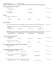### Commutative Property of Multiplication Commutative Property of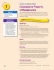### eureka - Innova Valencia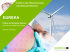### RANCANGAN PELAJARAN TAHUNAN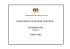### nike air force i - Projects at NFSTC.org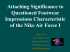### Catalogue Supplement 3 - minus AB 20mm.indd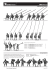### Pick-A-Mood Manual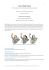### Changing Our Minds, Changing Their Lives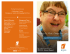### Agents With Faces - What Can We Learn From LEGO Minifigures?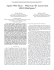### Introduction to Computational Fluid Dynamics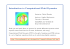### course description book - Bloomfield Hills Schools